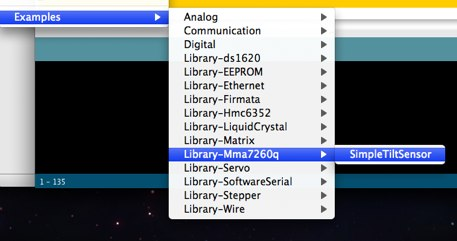# Ruben Laguna's blog

Mar 21, 2009 - 2 minute read - Comments - accelerometer arduino electronics library max756 mma7260q sensor tilt xbee

# Arduino library for MMA7260Q (Tilt Sensor)

In the past days I published a couple of arduino libraries. All coming from by XBee sensor development. I’m still waiting for the MAX756 to arrive from Futurlec, I need the MAX756 to power the xbee sensor with batteries and mount it in its final position. So in the meanwhile I’m cleaning a little bit the code, moving thing to libraries, etc. Now it’s the turn of the MMA7260Q, (see other posts about MMA7260q) .

I created an Arduino library to use MMA7260Q as a Tilt Sensor that does autozero calibration.

The library contains an example that can be accessed through the File ⇒ Sketchbook ⇒ Examples ⇒ Library-Mma7260q ⇒ SimpleTiltSensor.The example looks like this (also available as gist):

``````#include "mma7260q.h"

//set up MMA7260Q
Mma7260q mma7260q = Mma7260q(0,1,2);

long time = millis();

void setup() {

Serial.begin(9600);
delay(100);

//initial calibration of the MMA7260q
// you can get the correct values for your MMA7260q
// by running this program and copying the max and min values
// after rotating the mma7260q 360degrees around all axis
mma7260q.autoZeroCalibration(173,192,258);
mma7260q.autoZeroCalibration(766,720,914);

Serial.print("RST\r\n");delay(100);
}

void loop()
{
float rho, phi, theta;

if ((millis()-time)>2000)
{
time = millis();
Serial.print("rho : ");
printFloat(rho,3);
Serial.print(" phi : ");
printFloat(phi,3);
Serial.print(" the: ");
printFloat(theta,3);
Serial.print("\r\n");

int minx,miny,minz,maxx,maxy,maxz;
mma7260q.getMinValues(&minx,&miny,&minz);
mma7260q.getMaxValues(&maxx,&maxy,&maxz);
Serial.print("minx: ");
Serial.print((int)minx,DEC);
Serial.print(" miny: ");
Serial.print((int)miny,DEC);
Serial.print(" minz: ");
Serial.print((int)minz,DEC);
Serial.print("\r\n");

Serial.print("maxx: ");
Serial.print((int)maxx,DEC);
Serial.print(" maxy: ");
Serial.print((int)maxy,DEC);
Serial.print(" maxz: ");
Serial.print((int)maxz,DEC);
Serial.print("\r\n");

}
}

void printFloat(float value, int places) {
// this is used to cast digits
int digit;
float tens = 0.1;
int tenscount = 0;
int i;
float tempfloat = value;

// if value is negative, set tempfloat to the abs value
// make sure we round properly. this could use pow from
//<math.h>, but doesn't seem worth the import
// if this rounding step isn't here, the value 54.321 prints as
//54.3209

// calculate rounding term d: 0.5/pow(10,places)
float d = 0.5;
if (value < 0)
d *= -1.0;
// divide by ten for each decimal place
for (i = 0; i < places; i**)
d /= 10.0;
// this small addition, combined with truncation will round our
// values properly
tempfloat += d;

// first get value tens to be the large power of ten less than value
// tenscount isn't necessary but it would be useful if you wanted
// to know after this how many chars the number will take

if (value < 0)
tempfloat *= -1.0;
while ((tens * 10.0) <= tempfloat) {
tens *= 10.0;
tenscount += 1;
}

// write out the negative if needed
if (value < 0)
Serial.print('-');

if (tenscount == 0)
Serial.print(0, DEC);

for (i=0; i< tenscount; i**) {
digit = (int) (tempfloat/tens);
Serial.print(digit, DEC);
tempfloat = tempfloat - ((float)digit * tens);
tens /= 10.0;
}

// if no places after decimal, stop now and return
if (places <= 0)
return;

// otherwise, write the point and continue on
Serial.print('.');

// now write out each decimal place by shifting digits one by one
// into the ones place and writing the truncated value
for (i = 0; i < places; i**) {
tempfloat *= 10.0;
digit = (int) tempfloat;
Serial.print(digit,DEC);
// once written, subtract off that digit
tempfloat = tempfloat - (float) digit;
}``````

References: